# 视觉slam14讲——第10讲后端1

• 理解后端的概念
• 理解以EKF为代表的滤波器后端工作原理
• 理解非线性优化的后端，明白稀疏性是如何利用的
• 使用g2o和Ceres实际操作后端优化

# 1 概述

## 1.1 状态估计的概率解释

xk=f(xk1,μk)+ωk$x_k=f(x_{k-1},\mu_k)+\omega_k$
zk,j=h(yj,xk)+vk,j$z_{k,j}=h(y_j,x_k)+v_{k,j}$

• 只有当xk$x_k$观测到了yi$y_i$，才会产生观测数据。事实上在一个位置通常只能看到一小部分路标。
• 在没有运动方程的情况下，整个优化问题就只由许多个观测方程组成，类似于SfM（Structure from Motion）问题，相当于通过一组图像来恢复运动和结构。

P(xk|x0,μ1:k,z1:k1)=P(xk|xk1,x0,μ1:k,z1:k1)P(xk1|x0,μ1:k,z1:k1)dxk1

（1）假设马尔科夫性，使用EKF方法
（2）考虑k时刻的状态与之前说有的状态关系，使用非线性优化方法

## 1.2 线性系统和KF

P(xk|xk1,x0,μ1:k,z1:k1)=P(xk|xk1,μk)

P(xk1|x0,μ1:k,z1:k1)dxk1=P(xk1|x0,μ1:k1,z1:k1)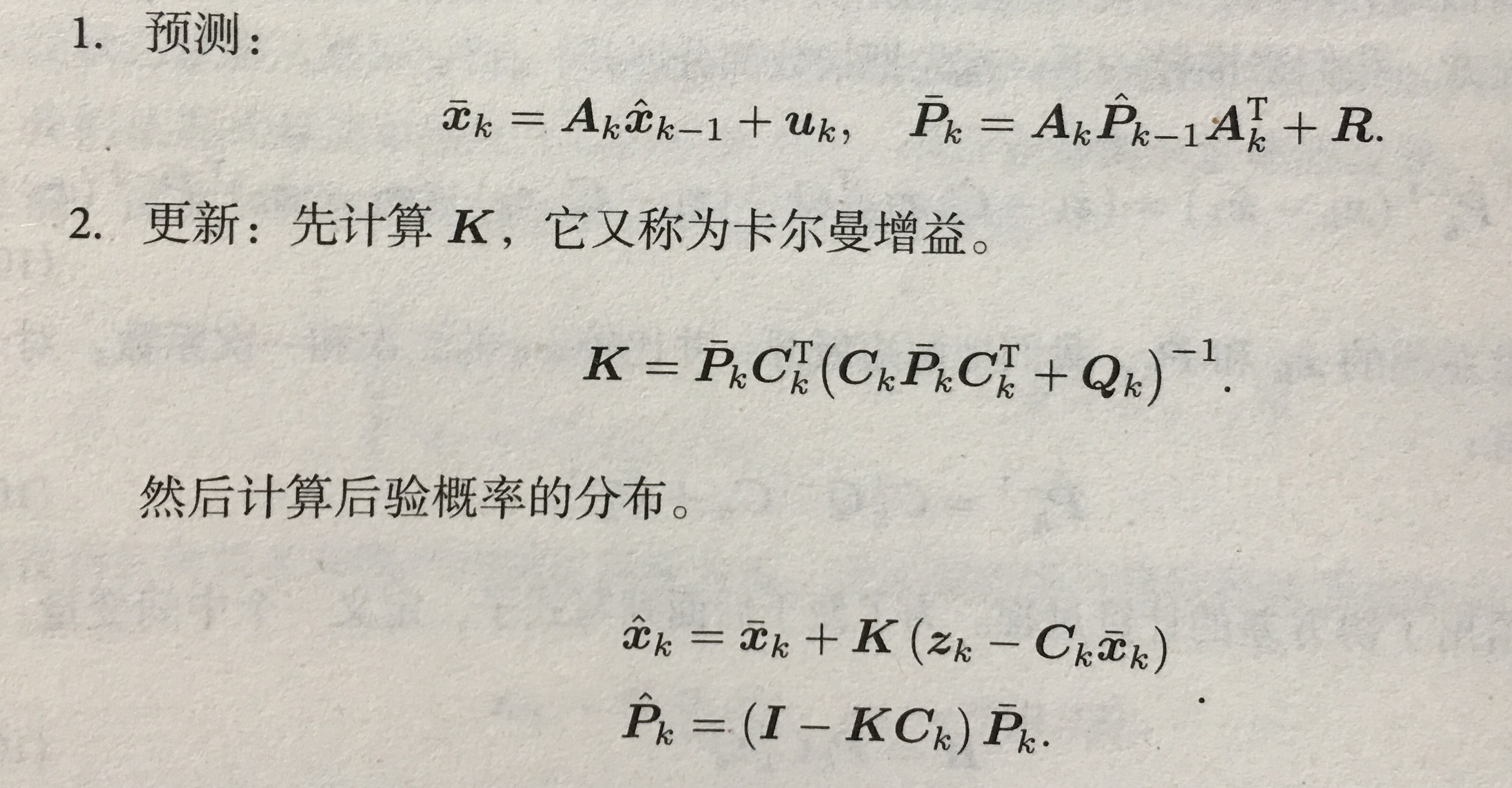## 1.3 非线性系统和EKF

SLAM中运动方程和观测方程通常是非线性函数，尤其是SLAM中的相机模型，需要使用相机内参模型和李代数表示的位姿，更不可能是线性系统。一个高斯分布，经过非线性变换后，也往往不再是高斯分布，所以在非线性系统中，必须取一定的近似，将一个非高斯分布近似为高斯分布。

# 2 BA与图优化

## 2.1 投影模型和BA代价函数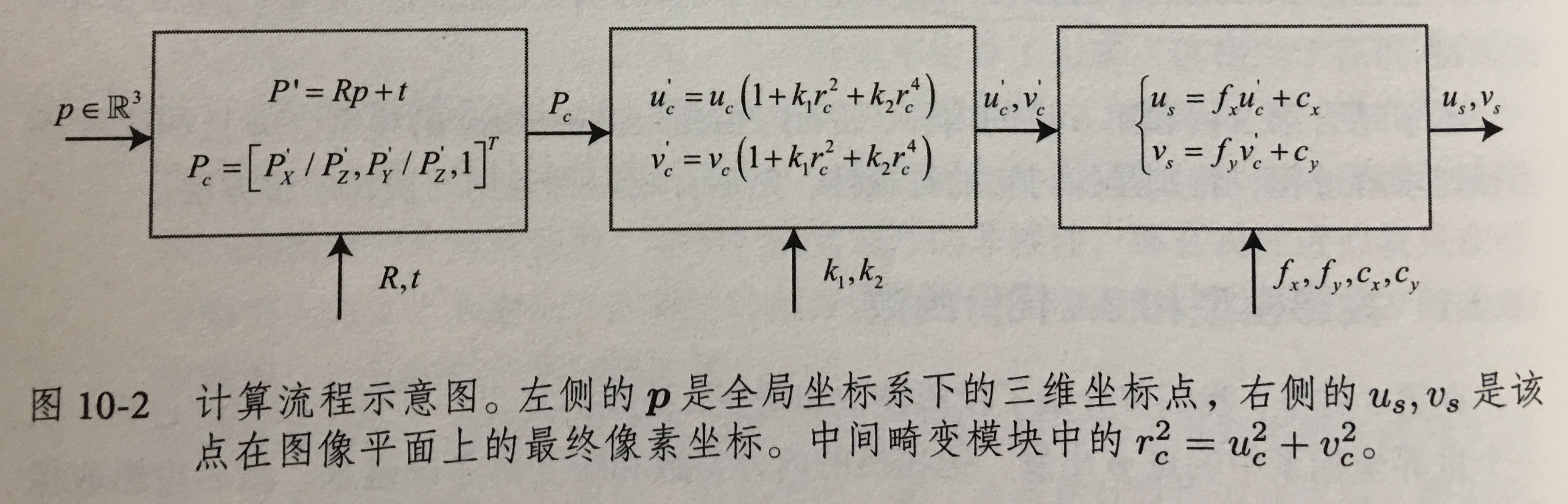12i=1mj=1n||eij||2=12i=1mj=1n||zijh(ξi,pj)||2

## 2.2 BA的求解

12||f(x+x)||2=12||e+Fxc+Exp||2

J=[FE]

H=JTJ=[FTFETFFTEETE]

## 2.3 稀疏性和边缘化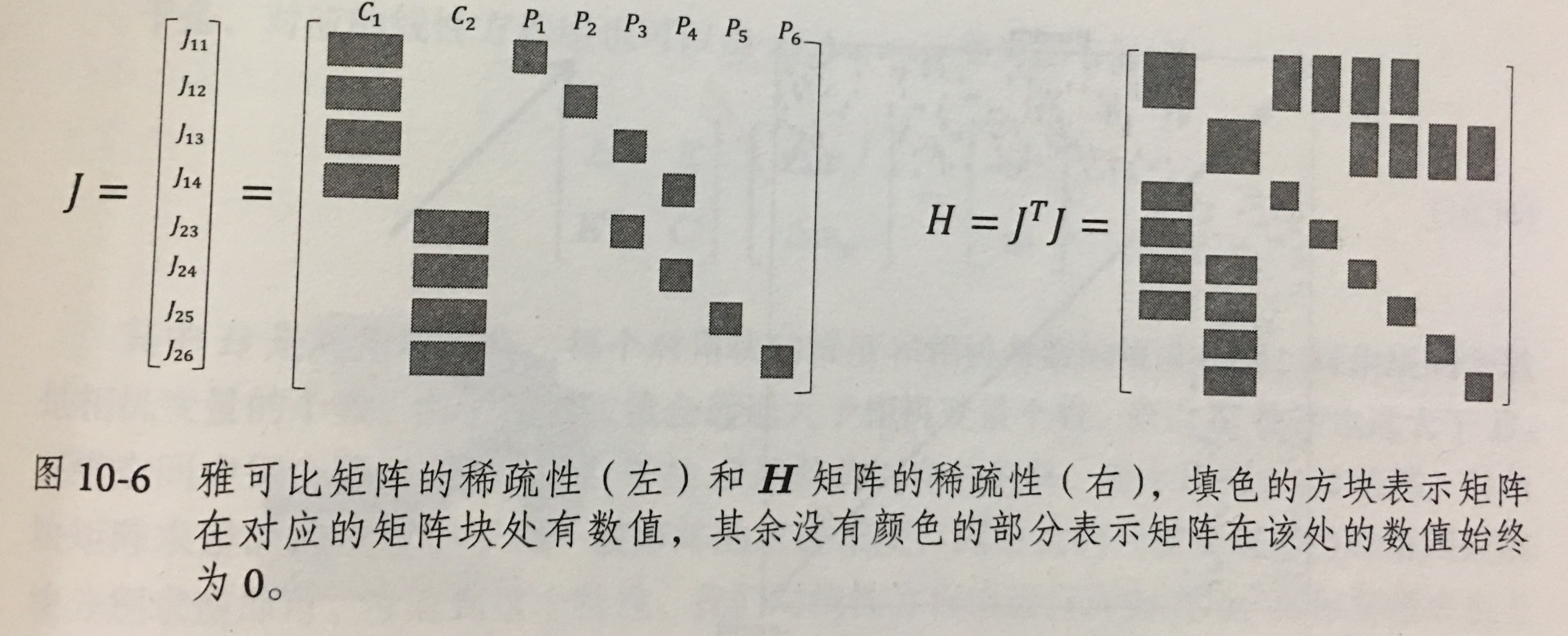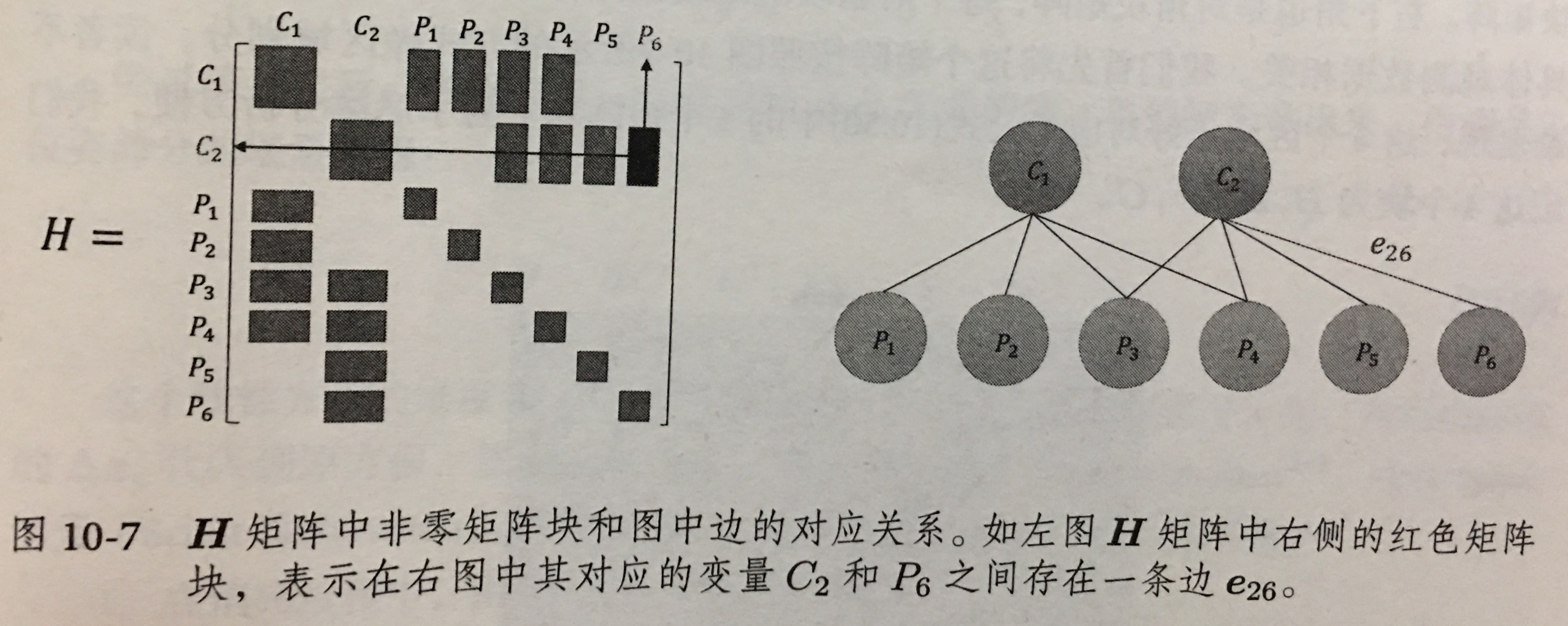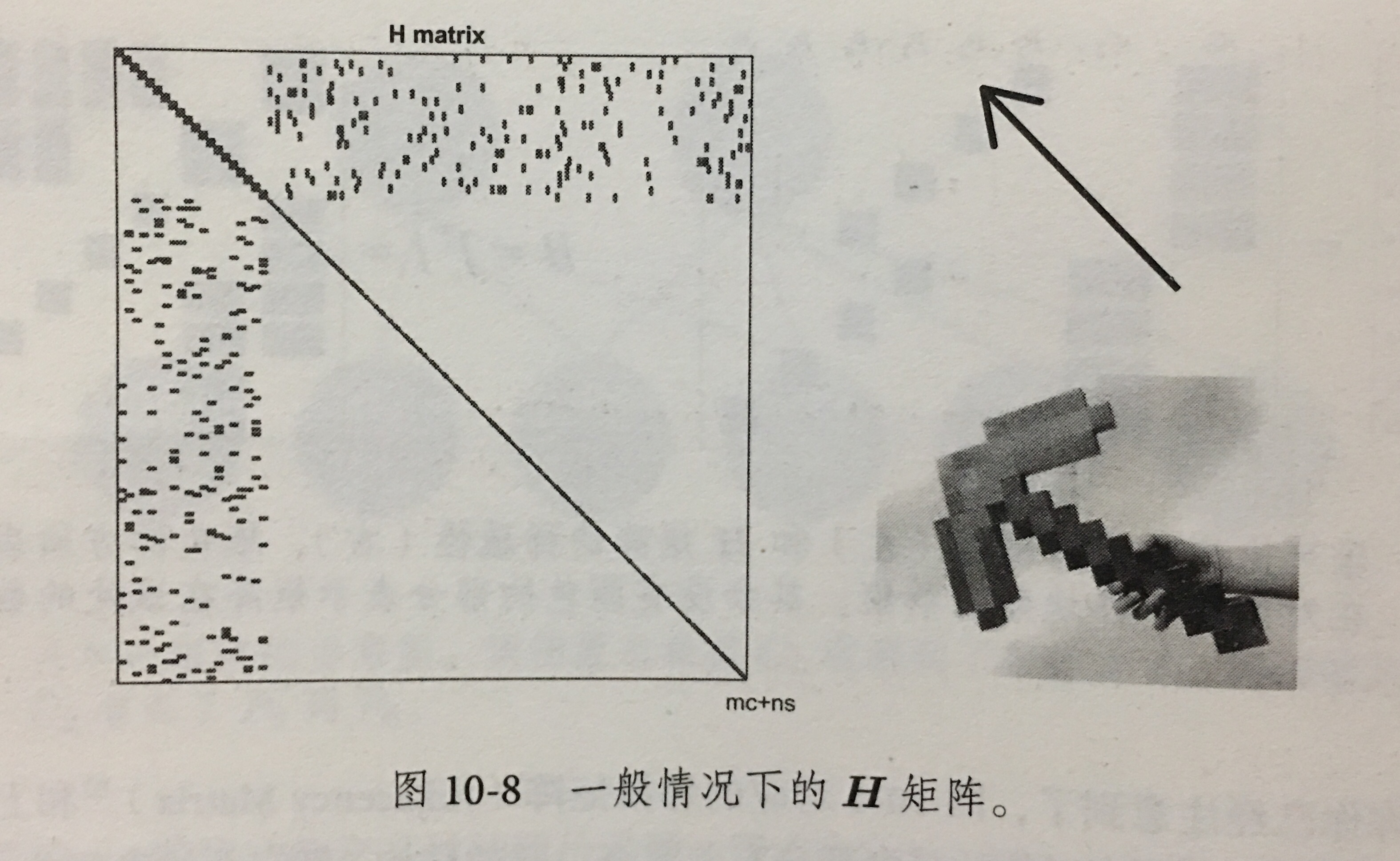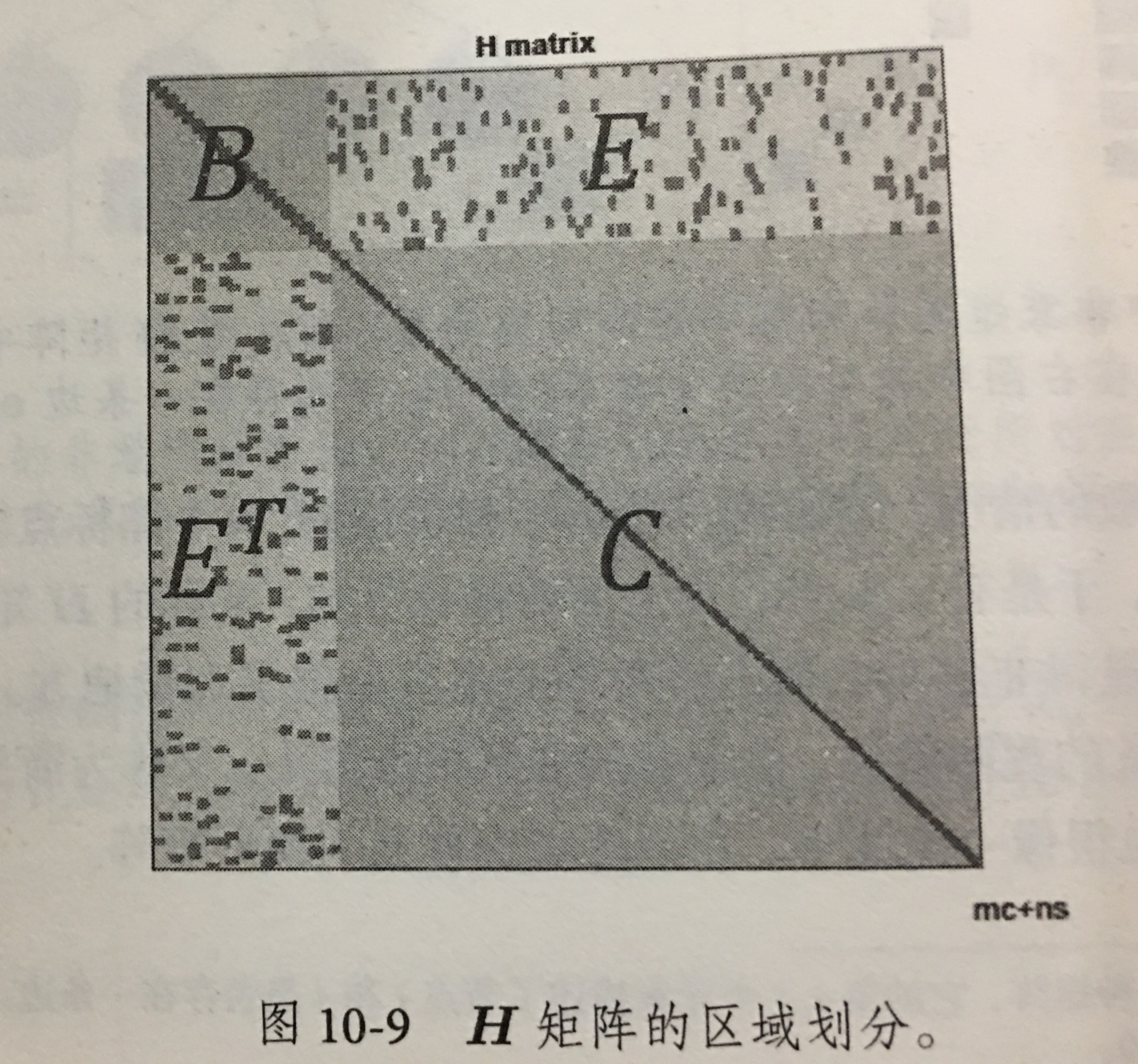[BEC1ET]xc=vEC1ω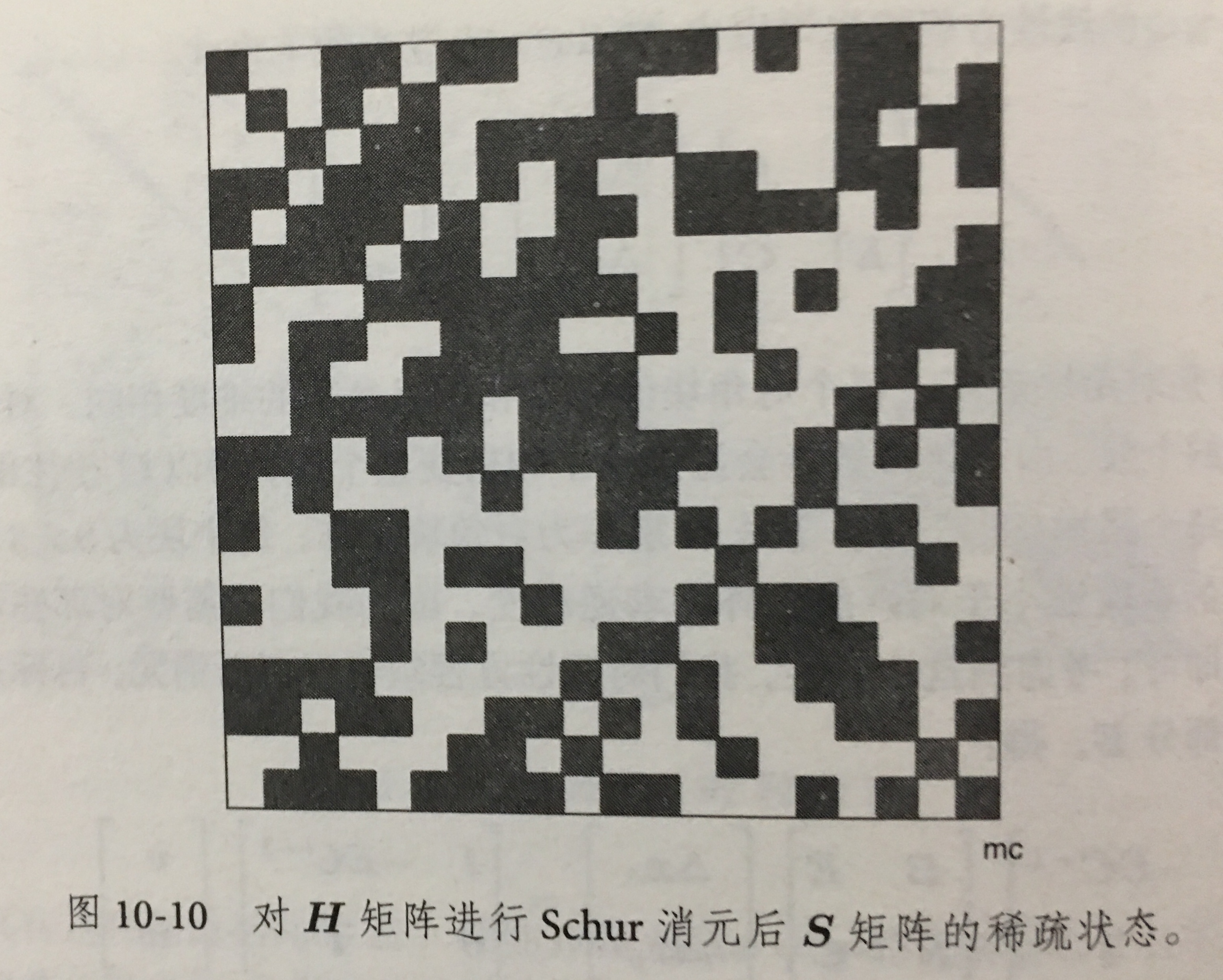H矩阵的非对角块处的非零元素对应着相机和路标的关联，进行Schur消元后的S的稀疏性具有物理意义，表明该处对应两个相机变量之间存在着共同观测的路标点，称为共视（Co-visibility）。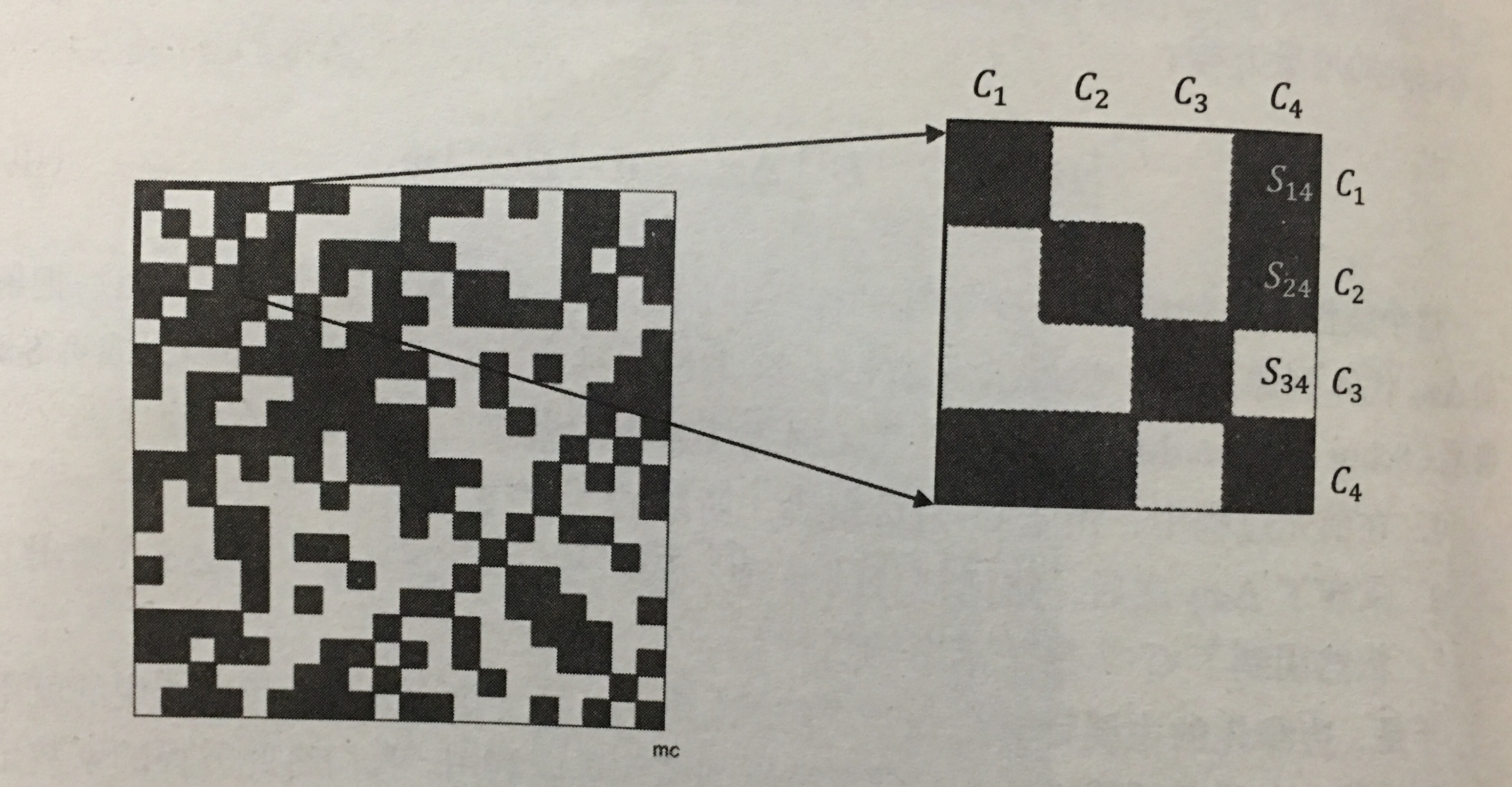S矩阵的稀疏性结构取决于实际的观测结果，无法提前预知。

©️2019 CSDN 皮肤主题: 大白 设计师: CSDN官方博客